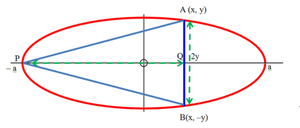Related Articles
Area of largest isosceles triangle that can be inscribed in an Ellipse whose vertex coincides with one extremity of the major axis
• Last Updated : 25 Mar, 2021

Given an ellipse with half the major and minor axes length A & B, the task is to find the area of the largest isosceles triangle that can be inscribed in the ellipse whose vertex coincides with one extremity of the major axis.

Examples:

Input: A = 1, B = 2
Output: 2.598
Explanation:
Area of the isosceles triangle = ((3 * √3) * A * B) / 4.
Therefore, area = 2.598.

Input: A = 2, B = 3
Output: 7.794

Approach: The idea is based on the following mathematical formula:

Proof:Considering triangle APB,
Area of APB = AB * PQ = (1 / 2) * A * B * (2 sin∅ – sin2∅)

Taking derivative:
d(area(APB))/d∅ = ab ( cos∅ – cos2∅)

Equating the derivative to zero:
d(area(APB))/d∅ = 0
cos∅ = – (1 / 2)
∅ = 2PI / 3

Therefore, area of APB = (3√3) * A * B / 4

Below is the implementation of the above approach:

## C++

 `// C++ program for the above approach` `#include ``using` `namespace` `std;` `// Function to calculate area``// of the isosceles triangle``void` `triangleArea(``float` `a, ``float` `b)``{``    ``// If a and b are negative``    ``if` `(a < 0 || b < 0) {``        ``cout << -1;``        ``return``;``    ``}` `    ``// Stores the area of the triangle``    ``float` `area = (3 * ``sqrt``(3) * a * b) / (4);` `    ``// Print the area``    ``cout << area;``}` `// Driver code``int` `main()``{``    ``// Given value of a & b``    ``float` `a = 1, b = 2;` `    ``// Function call to find the``    ``// area of the isosceles traingle``    ``triangleArea(a, b);` `    ``return` `0;``}`

## Java

 `// Java program for the above approach``import` `java.util.*;` `class` `GFG``{` `// Function to calculate area``// of the isosceles triangle``static` `void` `triangleArea(``float` `a, ``float` `b)``{``  ` `    ``// If a and b are negative``    ``if` `(a < ``0` `|| b < ``0``) {``        ``System.out.println(-``1``);``        ``return``;``    ``}` `    ``// Stores the area of the triangle``    ``float` `area = (``3` `* (``float``)Math.sqrt(``3``) * a * b) / (``4``);` `    ``// Print the area``    ``System.out.println(area);``}` `// Driver Code``public` `static` `void` `main(String[] args)``{``  ` `    ``// Given value of a & b``    ``float` `a = ``1``, b = ``2``;` `    ``// Function call to find the``    ``// area of the isosceles traingle``    ``triangleArea(a, b);``}``}` `// This code is contributed by sanjoy_62.`

## Python3

 `# Python 3 program for the above approach``from` `math ``import` `sqrt` `# Function to calculate area``# of the isosceles triangle``def` `triangleArea(a, b):``  ` `    ``# If a and b are negative``    ``if` `(a < ``0` `or` `b < ``0``):``        ``print``(``-``1``)``        ``return` `    ``# Stores the area of the triangle``    ``area ``=` `(``3` `*` `sqrt(``3``) ``*` `a ``*` `b) ``/` `(``4``);` `    ``# Print the area``    ``print``(``"{:.5f}"``.``format``(area))` `# Driver code``if` `__name__ ``=``=` `'__main__'``:``  ` `    ``# Given value of a & b``    ``a ``=` `1``    ``b ``=` `2``    ` `    ``# Function call to find the``    ``# area of the isosceles traingle``    ``triangleArea(a, b)``    ` `    ``# This code is contributed by SURENDRA_GANGWAR.`

## C#

 `// C# program for the above approach` `using` `System;``public` `class` `GFG``{` `  ``// Function to calculate area``  ``// of the isosceles triangle``  ``static` `void` `triangleArea(``float` `a, ``float` `b)``  ``{` `    ``// If a and b are negative``    ``if` `(a < 0 || b < 0) {``      ``Console.WriteLine(-1);``      ``return``;``    ``}` `    ``// Stores the area of the triangle``    ``float` `area = (3 * (``float``)Math.Sqrt(3) * a * b) / (4);` `    ``// Print the area``    ``Console.WriteLine(area);``  ``}` `  ``// Driver Code``  ``public` `static` `void` `Main(``string``[] args)``  ``{` `    ``// Given value of a & b``    ``float` `a = 1, b = 2;` `    ``// Function call to find the``    ``// area of the isosceles traingle``    ``triangleArea(a, b);``  ``}``}` `// This code is contributed by AnkThon`

## Javascript

 ``
Output:
`2.59808`

Time Complexity: O(1)
Auxiliary Space: O(1)

Attention reader! Don’t stop learning now. Get hold of all the important mathematical concepts for competitive programming with the Essential Maths for CP Course at a student-friendly price. To complete your preparation from learning a language to DS Algo and many more,  please refer Complete Interview Preparation Course.

My Personal Notes arrow_drop_up